Next: Polynomial division Up: DAMPED OSCILLATION Previous: DAMPED OSCILLATION

## Narrow-band filters

It seems we can represent a sinusoid by Z-transforms by setting a pole on the unit circle. Taking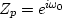, we have the filter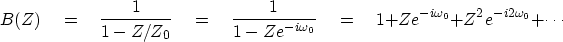(25)
The signal bt seems to be the complex exponential,but it is not quite that because bt is turned on'' at t = 0, whereasis nonzero at negative time.

Now, how can we make a real-valued sinusoid starting at t=0? Just as with zeros, we need to complement the pole atby one at.The resulting signal bt is shown on the left in Figure 7. On the right is a graphical attempt to plot the impulse function of dividing by zero at.sinus
Figure 7
A pole on the real axis (and its mate at negative frequency) gives an impulse function at that frequency and a sinusoidal function in time.

Next, let us look at a damped case like leaky integration. Letand.Then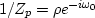.Define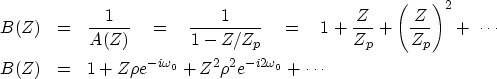(26) (27)
The signal bt is zero before t = 0 and is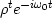after t = 0. It is a damped sinusoidal function with amplitude decreasing with time as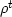.We can readily recognize this as an exponential decay(28)
where the approximation is best for values ofnear unity.

The wavelet bt is complex. To have a real-valued time signal, we need another pole at the negative frequency, say.So the composite denominator is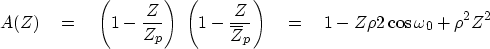(29)

Multiplying the two poles together as we did for roots results in the plots of 1/A(Z) in Figure 8.dsinus
Figure 8
A damped sinusoidal function of time transforms to a pole near the real-axis, i.e., just outside the unit circle in the Z-plane.

Notice the p'' in the figure. It indicates the location of the pole Zp but is shown in the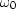-plane, where.Pushing the p'' left and right will lower and raise the resonant frequency. Pushing it down and up will raise and lower the duration of the resonance.

## EXERCISES:

1. How far from the unit circle are the poles of 1/(1 - .1Z + .9Z2)? What is the decay time of the filter and its resonant frequency?Next: Polynomial division Up: DAMPED OSCILLATION Previous: DAMPED OSCILLATION
Stanford Exploration Project
10/21/1998